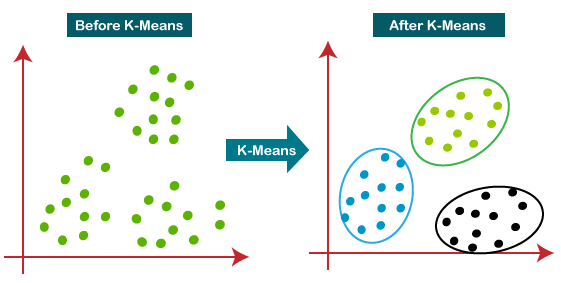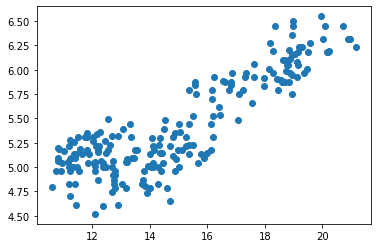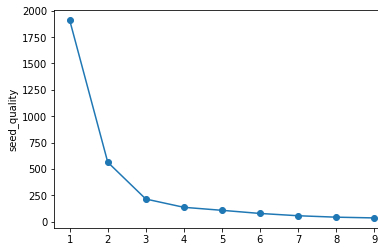• +91-9872993883
• +91-8283824812
• info@ris-ai.com
k-means clustering

# K-means Clustering in Machine Learning¶

Hello guys, in this note book we will learn about the K-mean Clustering in machine learning.what is k-means ,how it works and its implementation using python.So, let's begin with k-means.

So first question came in mind what is unsupervised learning? Unsupervised learning is a type of machine learning in which models are trained using unlabeled dataset and are allowed to act on that data without any supervision.

Now in K-means clustring A cluster refers to a collection of data points aggregated together because of certain similarities.

It is an iterative algorithm that divides the unlabeled dataset into k different clusters in such a way that each dataset belongs only one group that has similar properties.

## How does the K-Means Algorithm Work?¶

The algorithm takes the unlabeled dataset as input, divides the dataset into k-number of clusters, and repeats the process until it does not find the best clusters. The value of k should be predetermined in this algorithm.

The k-means clustering algorithm mainly performs two tasks:

1. Determines the best value for K center points or centroids by an iterative process.
2. Assigns each data point to its closest k-center. Those data points which are near to the particular k-center, create a cluster.

Hence each cluster has datapoints with some commonalities, and it is away from other clusters.### Steps to perform K-Means ¶

The working of the K-Means algorithm is explained in the below steps:

Step-1: Select the number K to decide the number of clusters.

Step-2: Select random K points or centroids. (It can be other from the input dataset).

Step-3: Assign each data point to their closest centroid, which will form the predefined K clusters.

Step-4: Calculate the variance and place a new centroid of each cluster.

Step-5: Repeat the third steps, which means reassign each datapoint to the new closest centroid of each cluster.

Step-6: If any reassignment occurs, then go to step-4 else go to FINISH.

#### Python Implementation of K-means Clustering Algorithm ¶

Before implementation, let's understand what type of problem we will solve here. So, we have a dataset of seeds quality, which tells about to which group seeds belong to.

In the given dataset, we have area,perimeter,compactness, lengthOfKernel, widthOfKernel,widthOfKernel gro ,lengthOfKernelGroove and seed_quality . From this dataset, we need to calculate some patterns, as it is an unsupervised method, so we don't know what to calculate exactly.

### Data pre-processing Step ¶

The first step will be the data pre-processing, as we did in our earlier topics of Regression and Classification. But for the clustering problem, it will be different from other models. Let's discuss it:

a) Importing Libraries

As we did in previous topics, firstly, we will import the libraries for our model, which is part of data pre-processing. The code is given below:

In the above code, the numpy we have imported for the performing mathematics calculation, matplotlib is for plotting the graph, and pandas are for managing the dataset.

In :
import pandas as pd
import numpy as np
from sklearn.cluster import KMeans
from matplotlib import pyplot as plt
%matplotlib inline


b)Importing the Dataset:

Next, we will import the dataset that we need to use. So here, we are using the seeds_dataset.csv dataset. It can be imported using the below code:

In :
df=pd.read_csv("seeds_dataset.csv")


Out:
area perimeter compactness lengthOfKernel widthOfKernel widthOfKernel gro lengthOfKernelGroove seed_quality
0 15.26 14.84 0.8710 5.763 3.312 2.221 5.220 1
1 14.88 14.57 0.8811 5.554 3.333 1.018 4.956 1
2 14.29 14.09 0.9050 5.291 3.337 2.699 4.825 1
3 13.84 13.94 0.8955 5.324 3.379 2.259 4.805 1
4 16.14 14.99 0.9034 5.658 3.562 1.355 5.175 1

# K-means Clustering in Machine Learning ¶

### Visualization of whole data ¶

In :
plt.scatter(df['area'],df['lengthOfKernelGroove'])

Out:
<matplotlib.collections.PathCollection at 0x7fc19ed0c6a0>### Using K-means algorithm on traning dataset ¶

As we have got the number of clusters, so we can now train the model on the dataset. we will use 4, as we know there are 4clusters that need to be formed. The code is given below:

In :
km=KMeans(n_clusters=4)
km

Out:
KMeans(n_clusters=4)
In :
y_pre=km.fit_predict(df[['area','lengthOfKernelGroove']])
y_pre

Out:
array([3, 2, 2, 2, 3, 2, 2, 2, 3, 3, 3, 2, 2, 2, 2, 2, 2, 3, 2, 0, 2, 2,
3, 0, 2, 3, 2, 0, 2, 2, 2, 3, 2, 2, 2, 3, 3, 3, 2, 2, 2, 2, 2, 3,
2, 2, 3, 2, 2, 2, 2, 3, 2, 2, 2, 2, 2, 2, 3, 0, 0, 0, 0, 2, 0, 0,
2, 2, 2, 0, 3, 3, 3, 1, 3, 3, 3, 1, 1, 3, 3, 1, 1, 1, 1, 1, 1, 1,
1, 1, 1, 1, 1, 1, 1, 3, 1, 1, 1, 1, 3, 1, 1, 1, 1, 1, 1, 3, 1, 1,
1, 1, 1, 1, 1, 1, 1, 1, 1, 1, 1, 1, 3, 1, 3, 1, 1, 1, 1, 3, 1, 1,
3, 3, 3, 3, 3, 3, 3, 3, 2, 2, 2, 0, 0, 0, 0, 0, 0, 0, 0, 0, 0, 0,
0, 0, 0, 0, 0, 0, 0, 0, 0, 0, 0, 0, 0, 0, 0, 0, 0, 0, 0, 0, 0, 0,
0, 0, 0, 0, 0, 0, 0, 0, 0, 0, 0, 0, 0, 0, 0, 0, 0, 0, 0, 0, 0, 2,
0, 0, 0, 0, 0, 0, 0, 0, 0, 2, 0, 0], dtype=int32)
In :
df['cluster']=y_pre
df

Out:
area perimeter compactness lengthOfKernel widthOfKernel widthOfKernel gro lengthOfKernelGroove seed_quality cluster
0 15.26 14.84 0.8710 5.763 3.312 2.221 5.220 1 3
1 14.88 14.57 0.8811 5.554 3.333 1.018 4.956 1 2
2 14.29 14.09 0.9050 5.291 3.337 2.699 4.825 1 2
3 13.84 13.94 0.8955 5.324 3.379 2.259 4.805 1 2
4 16.14 14.99 0.9034 5.658 3.562 1.355 5.175 1 3
... ... ... ... ... ... ... ... ... ...
205 12.19 13.20 0.8783 5.137 2.981 3.631 4.870 3 0
206 11.23 12.88 0.8511 5.140 2.795 4.325 5.003 3 0
207 13.20 13.66 0.8883 5.236 3.232 8.315 5.056 3 2
208 11.84 13.21 0.8521 5.175 2.836 3.598 5.044 3 0
209 12.30 13.34 0.8684 5.243 2.974 5.637 5.063 3 0

210 rows × 9 columns

In :
km.cluster_centers_

Out:
array([[11.83893333,  5.05990667],
[19.15104167,  6.12725   ],
[14.1277551 ,  5.09177551],
[16.27763158,  5.59465789]])

### Visualizing the Clusters ¶

The last step is to visualize the clusters. As we have 4 clusters for our model, so we will visualize each cluster one by one.

To visualize the clusters will use scatter plot using plt.scatter() function of matplotlib.

In :
df1=df[df.cluster==0] #we save each cluster in new dataframe
df2=df[df.cluster==1]
df3=df[df.cluster==2]
df4=df[df.cluster==3]

plt.scatter(df1['area'],df1['lengthOfKernelGroove'],color='yellow',marker='o')
plt.scatter(df4['area'],df4['lengthOfKernelGroove'],color='blue',marker='o')
plt.scatter(df2['area'],df2['lengthOfKernelGroove'],color='red',marker='o')
plt.scatter(df3['area'],df3['lengthOfKernelGroove'],color='green',marker='o')
plt.scatter(km.cluster_centers_[:,0],km.cluster_centers_[:,1],color='black',marker='^',label='centroid',s = 300)
plt.xlabel('area')
plt.ylabel('lengthOfKernelGroove')
plt.legend()

Out:
<matplotlib.legend.Legend at 0x7fc19696fbe0>In :
krange=range(1,10)
sse=[] #sum of square error array
for k in krange:
km=KMeans(n_clusters=k) #we are making kmean models of each cluster and storing k cluster
km.fit(df[['area','seed_quality']])
sse.append(km.inertia_) #we get sumof square error from inertia


### The elbow method ¶

The elbow method runs k-means clustering on the dataset for a range of values for k (say from 1-10) and then for each value of k computes an average score for all clusters. By default, the distortion score is computed, the sum of square distances from each point to its assigned center.

The “elbow” method help data scientists to select the optimal number of clusters by fitting the model with a range of values for 𝐾. If the line chart resembles an arm, then the “elbow” (the point of inflection on the curve) is a good indication that the underlying model fits best at that point.

In :
plt.xlabel('area')
plt.ylabel('seed_quality')
plt.plot(krange,sse,marker='o')

Out:
[<matplotlib.lines.Line2D at 0x7fd668289048>]In [ ]:


In [ ]: